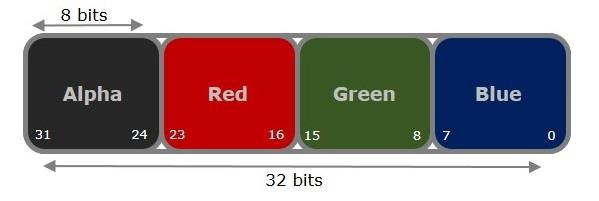# How to get pixels (RGB values) of an image using Java OpenCV library?

A digital image is stored as a 2D array of pixels and a pixel is the smallest element of a digital image.

Each pixel contains the values of alpha, red, green, blue values and the value of each color lies between 0 to 255 which consumes 8 bits (2^8).

The ARGB values are stored in 4 bytes of memory in the same order (right to left) with blue value at 0-7 bits, Green value at 8-15 bits, Red value at 16-23 bits and, alpha at 24-31 bits.## Retrieving the pixel contents (ARGB values) of an image −

To get the pixel values from an image −

• Loop through each pixel in the image. i.e. run nested loops traversing the height and width of the image.

• Get the pixel value at every point using the getRGB() method.

• Instantiate the Color object by passing the pixel value as a parameter.

• Get the Red, Green, Blue values using the getRed(), getGreen() and getBlue() methods respectively.

## Example

Following java, example reads the contents of every pixel of an image and writes the RGB values to a file −

import java.io.File;
import java.io.FileWriter;
import java.awt.Color;
import java.awt.image.BufferedImage;
import javax.imageio.ImageIO;
public class GetPixels {
public static void main(String args[])throws Exception {
FileWriter writer = new FileWriter("D:\Images\pixel_values.txt");
File file= new File("D:\Images\cat.jpg");
for (int y = 0; y < img.getHeight(); y++) {
for (int x = 0; x < img.getWidth(); x++) {
//Retrieving contents of a pixel
int pixel = img.getRGB(x,y);
//Creating a Color object from pixel value
Color color = new Color(pixel, true);
//Retrieving the R G B values
int red = color.getRed();
int green = color.getGreen();
int blue = color.getBlue();
writer.append(red+":");
writer.append(green+":");
writer.append(blue+"");
writer.append("\n");
writer.flush();
}
}
writer.close();
System.out.println("RGB values at each pixel are stored in the specified file");
}
}

## Output

RGB values at each pixel are stored in the specified file

You can also retrieve the RGB values from a pixel using the shift operators −

To do so −

• Right, shift to the beginning position of each color i.e. 24 for alpha 16 for red, etc.

• The shift right operation may impact the values of other channels, to avoid this, you need to perform bitwise and operation with 0Xff. This masks the variable leaving the last 8 bits and ignoring all the rest of the bits.

int p = img.getRGB(x, y);
//Getting the A R G B values from the pixel value
int a = (p>>24)&0xff;
int r = (p>>16)&0xff;
int g = (p>>8)&0xff;
int b = p&0xff;